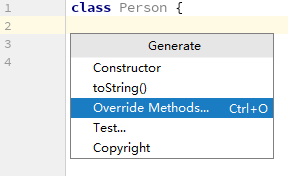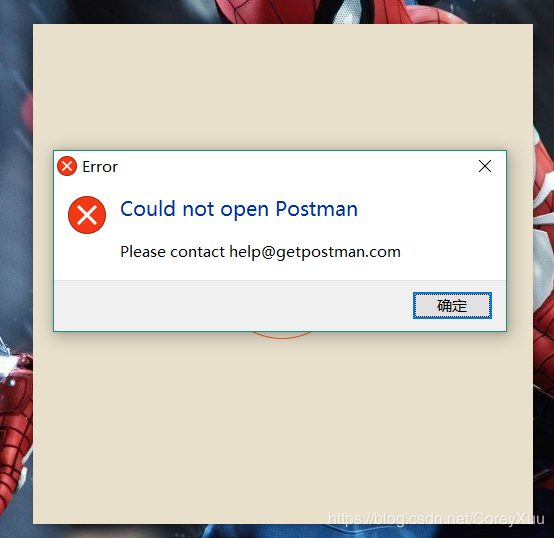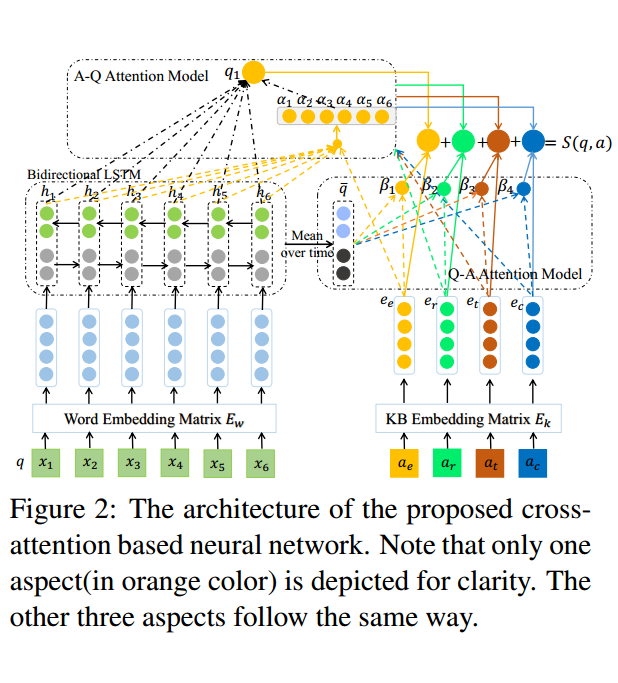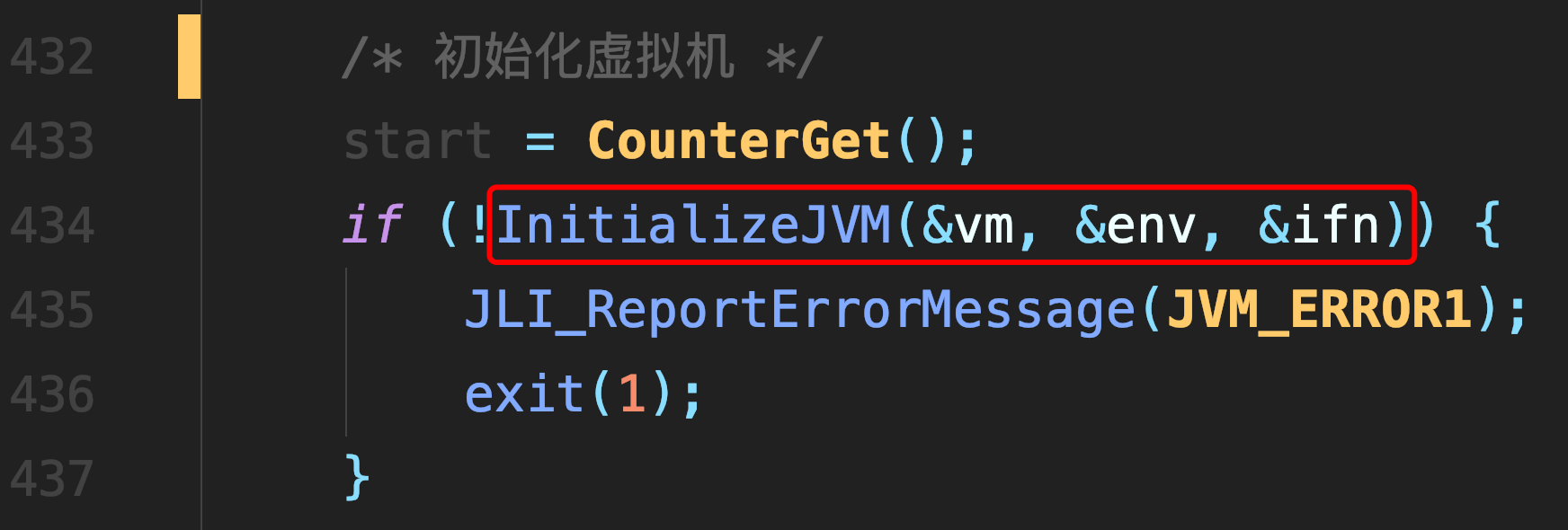# LeetCode-Longest Increasing Path in a Matrix

Given an integer matrix, find the length of the longest increasing path.

From each cell, you can either move to four directions: left, right, up or down. You may NOT move diagonally or move outside of the boundary (i.e. wrap-around is not allowed).

Example 1:

```nums = [
[9,9,4],
[6,6,8],
[2,1,1]
]
```

Return `4`
The longest increasing path is `[1, 2, 6, 9]`.

Example 2:

```nums = [
[3,4,5],
[3,2,6],
[2,2,1]
]
```

Return `4`
The longest increasing path is `[3, 4, 5, 6]`. Moving diagonally is not allowed.

Analysis:

We can use DFS + memrization.

At curret point (i,j), we detect all for directions, find the LIP of the 4 neighbor nodes and select the longest one to construct LIP of (i,j). Once we finish, we can record the LIP len of (i,j), so if this len is queried later, we do not need to find it again.

Some notes:

1. We do not need to record points on current search path, because it is increasing path, no furture point will be one of the points on path.

2. We do not need to record the points on the LIP of (i,j), intead, we just need to record the length.

3. We do not need to worry that a neighbor point's LIP will overlap with the points on current search path, because it is increasing path.

Solution:

``` 1 public class Solution {
2     int[] xMove = new int[]{1,0,-1,0};
3     int[] yMove = new int[]{0,1,0,-1};
4
5     public int longestIncreasingPath(int[][] matrix) {
6         if (matrix.length == 0 || matrix.length == 0)
7             return 0;
8
9         int[][] lipLen = new int[matrix.length][matrix.length];
10         int maxLen = 0;
11         for (int i = 0; i < matrix.length; i++)
12             for (int j = 0; j < matrix.length; j++) {
13                 int len = findLIPRecur(matrix, lipLen, i, j);
14                 if (maxLen < len)
15                     maxLen = len;
16             }
17
18         return maxLen;
19     }
20
21     public int findLIPRecur(int[][] matrix, int[][] lipLen, int x, int y) {
23         if (lipLen[x][y] != 0)
24             return lipLen[x][y];
25
26         int maxLen = 0;
27         for (int i = 0; i < xMove.length; i++) {
28             int nextX = x + xMove[i];
29             int nextY = y + yMove[i];
30             if (isFeasible(matrix, nextX, nextY) && matrix[nextX][nextY] > matrix[x][y]) {
31                 int len = findLIPRecur(matrix, lipLen, nextX, nextY);
32                 if (maxLen < len) {
33                     maxLen = len;
34                 }
35             }
36         }
37
38         // Record LIP len of current point
39         lipLen[x][y] = maxLen + 1;
40         return maxLen + 1;
41     }
42
43     public boolean isFeasible(int[][] matrix, int x, int y) {
44         return (x < matrix.length && x >= 0) && (y < matrix.length && y >= 0);
45     }
46
47
48 }```

##### 热门推荐
•浏览(654)
•浏览(563)
•浏览(547)
•浏览(480)
•浏览(424)
•浏览(419)
•浏览(381)
•浏览(366)
•浏览(362)
•浏览(344)